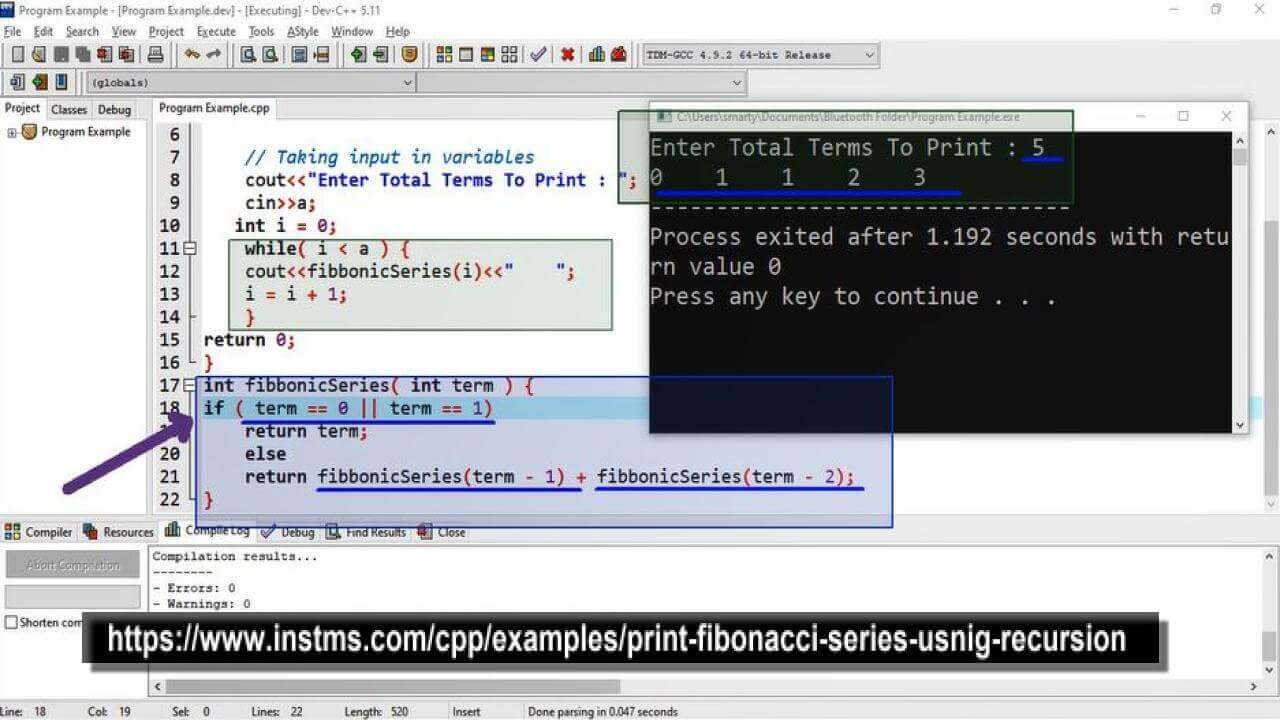# Print Fibonacci Series Usnig Recursion in CPP

## Source Code

#include <iostream>

using namespace std;

int fibbonicSeries( int );

int main(int argc, char** argv) {

int a;  // variable declaration for input

// Taking input in variables
cout<<"Enter Total Terms To Print : ";
cin>>a;

int i = 0;
while( i < a ) {
cout<<fibbonicSeries(a);

i = i + 1;
}

return 0;
}

int fibbonicSeries( int term ) {

if ( term == 0 || term == 1 )
return term;

return fibbonicSeries(term - 1) + fibbonicSeries(term - 2);
}

## Output## Working

This program take user input for printing fibonacci terms. It will print all terms using recursive function. In fibonacci series the first two terms are fixed so that these are used as base condition. Means when the provided term is 0 or 1 then function will not put a recursive call, otherwise a recursive call will be generated.

This recursive call will pass two parameters as per defination of fibonacci series that next generated number will be the sum of previous two numbers.